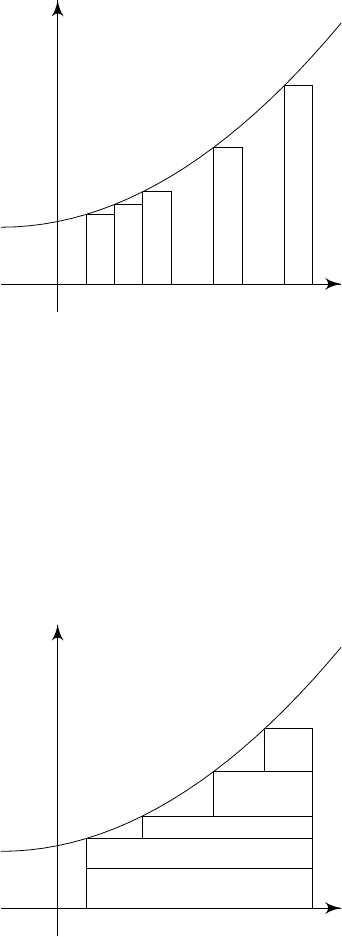0Introduction

II Probability and Measure0 Introduction
In measure theory, the main idea is that we want to assign “sizes” to different
sets. For example, we might think [0
,
2]
R
has size 2, while perhaps
Q R
has
size 0. This is known as a measure. One of the main applications of a measure
is that we can use it to come up with a new definition of an integral. The idea
is very simple, but it is going to be very powerful mathematically.
Recall that if
f
: [0
,
1]
R
is continuous, then the Riemann integral of
f
is
defined as follows:
(i) Take a partition 0 = t
0
< t
1
< ··· < t
n
= 1 of [0, 1].
(ii) Consider the Riemann sum
n
X
j=1
f(t
j
)(t
j
t
j1
)
(iii) The Riemann integral is
Z
f = Limit of Riemann sums as the mesh size of the partition 0.
x
y
0
t
1
t
2
t
3
···
t
k
t
k+1
···
···
1
The idea of measure theory is to use a different approximation scheme. Instead
of partitioning the domain, we partition the range of the function. We fix some
numbers r
0
< r
1
< r
2
< ··· < r
n
.
We then approximate the integral of f by
n
X
j=1
r
j
· (“size of f
1
([r
j1
, r
j
])”).
We then define the integral as the limit of approximations of this type as the
mesh size of the partition 0.
x
y
We can make an analogy with bankers If a Riemann banker is given a stack
of money, they would just add the values of the money in order. A measure-
theoretic banker will sort the bank notes according to the type, and then find
the total value by multiplying the number of each type by the value, and adding
up.
Why would we want to do so? It turns out this leads to a much more
general theory of integration on much more general spaces. Instead of integrating
functions [
a, b
]
R
only, we can replace the domain with any measure space.
Even in the context of
R
, this theory of integration is much much more powerful
than the Riemann sum, and can integrate a much wider class of functions. While
you probably don’t care about those pathological functions anyway, being able
to integrate more things means that we can state more general theorems about
integration without having to put in funny conditions.
That was all about measures. What about probability? It turns out the
concepts we develop for measures correspond exactly to many familiar notions
from probability if we restrict it to the particular case where the total measure
of the space is 1. Thus, when studying measure theory, we are also secretly
studying probability!